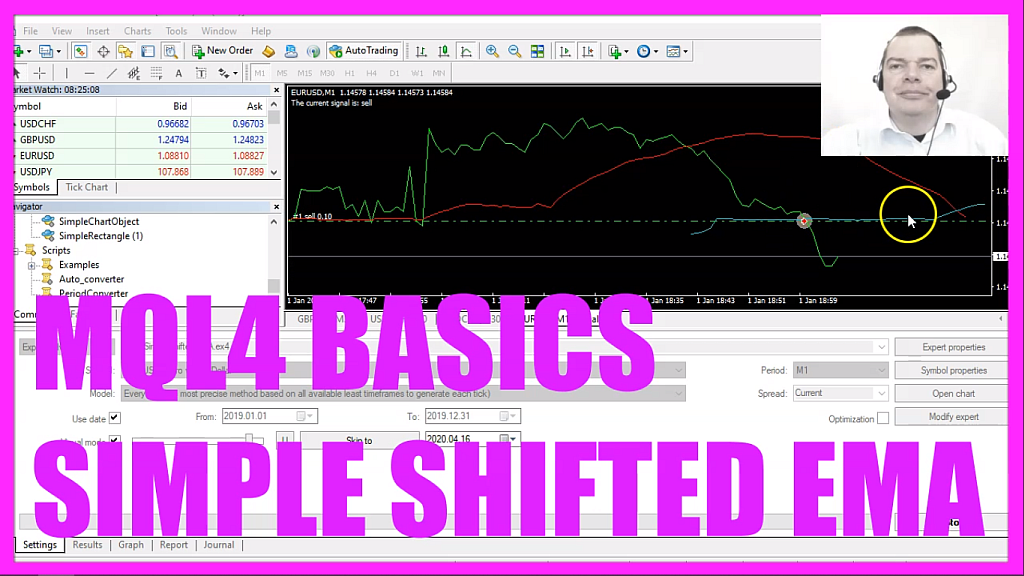In this video, we are going to create an expert advisor that is able to calculate buy and sell signals based on two shifted moving averages, so let’s find out how to do that with mql4.
To get started please click on a little icon here or press F4, now you should see the Metaeditor window and here you want to click on file, new file, expert advisor from template, continue, I will call this file simple shifted ema, click on continue, continue and finish.
Now you can delete everything above the ontick function and the two comment lines here, we start with a few user-defined variables, these are input variables, if you mark that and press F1 you will learn that these are variables that can be changed while the program is running, you don’t have to recompile the code, you can change the settings inside of the inputs tab.
The first value is for the number of candles that we are going to use to calculate the exponential moving average, the second one is a shift value, that’s twenty and we have another shift value one hundred and what those shift areas do is they move the moving average to the right side ahead of the price movement. When we zoom into the chart you can see that we have two moving averages that are similar but they are shifted to the right side, the first one is shifted twenty candles into the future and the second one one hundred candles into the future.
Inside of the on tick function, we create a string variable for the signal but we don’t assign any values here because we are going to calculate them now.
Let’s start with the first moving average. We use the included ima function that comes with mql4 for the current symbol on the chart and the currently selected period on that chart, we use the number of candles that we have defined here so this one will be for twenty candles, the next one is the shift value that we have defined here, that’s also for twenty candles. We are going to use mode ema that stands for exponential moving average, the values should be calculated based on the close price and we are always going to calculate the current candle value.
Well, if you mark the ima function and press F1 you will see that we have two shift values, this one, and this one that might look a little bit confusing but it’s not so hard to understand. This one is where you draw the moving average so in our case we draw it on the right side of the chart and this shift value here is for the candle number that should be calculated and we are going to calculate the current candle number so we are using a shift value of zero for this parameter.
Let’s repeat the whole process but this time we are going to calculate it for the second moving average but this one will use the value shift two and that is one hundred candles and that’s why it is moved far to the right side ahead of the price movement.
We want to buy if the price is above both ema’s so if the bit price is bigger than the first moving average and if the bid price is bigger than the second moving average that’s when we want to buy so now we assign the word buy to our signal. In the other case if the current bid price is below the first moving average and if the bid price is also below the second moving average that is when we want to sell and if this is true we are going to assign the word sell to our signal.
When our signal equals buy and the return value for orders total is zero that would mean we have no open orders and a buy signal and in that case, we use order send to buy ten micro lot.
Otherwise, if the signal equals sell and we have no open orders we use order send to sell ten micro lot.
Finally, we want to add a comment statement that should output the text, the current signal is, followed by the calculated signal.